Example 1: Stress on soil under a rectangular loaded area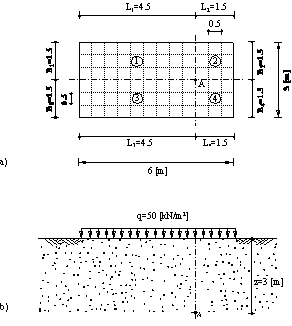Description of the problem

To verify the vertical stress at any point A below a rectangular loaded area, the stress on soil obtained by Das (1983) (Example 6.3, page 370) using influence coefficients of Newmark (1935) are compared with that obtained by ELPLA.

A distributed load of q = 50 [kN/m2] acts on a flexible rectangular area 6 [m] × 3 [m] as shown in Figure 1. It is required to determine the vertical stress at a point A, which is located at a depth of z = 3 [m] below the ground surface.Example 1: Stress on soil under a rectangular loaded area

Example 2: Stress on soil under a circular loaded area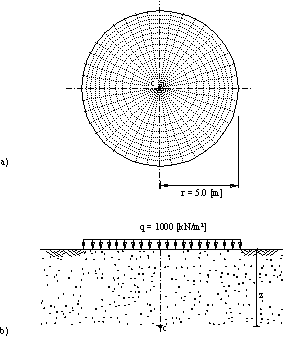Description of the problem

To verify the vertical stress at point c below the center of a circular loaded area, the influence coefficients of stress Iz below the center of a uniformly loaded area at the surface obtained by Scott (1974) (Table 12.2, page 287) are compared with those obtained by ELPLA.

Figure 2 shows a distributed load of q = 1000 [kN/m2] acts on a flexible circular area of radius r = 5 [m]. It is required to determine the vertical stress under the center c of the area at different depths z up to 10 [m] below the ground surface.Example 2: Stress on soil under a circular loaded area

Example 3: Immediate settlement under a loaded area on Isotropic elastic-half space mediumDescription of the problem

To verify the mathematical model of ELPLA for computing the immediate (elastic) settlement under a loaded area on Isotropic elastic-half space medium, the results of immediate settlement calculations obtained by Bowles (1977) (Table 5-4, page 157) are compared with those obtained by ELPLA.

The vertical displacement s under an area carrying a uniform pressure p on the surface of Isotropic elastic-half space medium can be expressed as............Example 3: Immediate settlement under a loaded area on Isotropic elastic-half space medium

Example 4: Immediate settlement under a rectangular loaded area on layered subsoil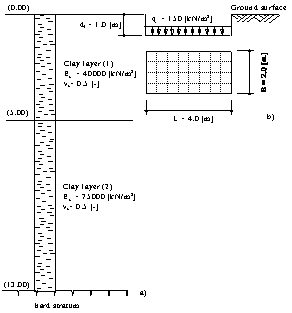Description of the problem

To verify the mathematical model of ELPLA for computing the immediate (elastic) settlement under a rectangular loaded area on layered subsoil, the immediate settlement of saturated clay layers under a rectangular loaded area calculated by Graig (1978) (Example 6.4, page 175) is compared with that obtained by ELPLA.

Janbu/ Bjerrum/ Kjaernsli (1956) presented a solution for the average settlement under an area carrying a uniform pressure q [kN/m2] on the surface of a limited soil layer using dimensionless factors. Factors are determined for Poisson’s ratio equal to νs = 0.5 [-].Example 4: Immediate settlement under a rectangular loaded area on layered subsoil

Example 5: Immediate settlement under a circular tank on layered subsoil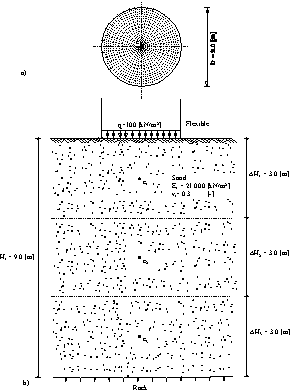Description of the problem

To verify the immediate settlement under a circular loaded area calculated by ELPLA, the immediate settlement at the center of a tank calculated by Das (1983) (Example 6.2, page 354) is compared with that obtained by ELPLA.

A circular tank of 3.0 [m] diameter is considered as shown in Figure 5. The base of the tank is assumed to be flexible and having a uniform contact pressure of q = 100 [kN/m2]. A sand layer 9.0 [m] thick is located under the tank. The modulus of elasticity of the sand is Es = 21000 [kN/m2] while Poisson’s ratio of the sand is ν = 0.3 [-].

It is required to determine the immediate settlement at the center of the tank for two cases:

a) Considering the underlying soil as one layer of 9.0 [m] thickness.

b) Dividing the underlying soil into three layers of equal thickness of 3.0 [m]

Example 6: Consolidation settlement under a rectangular raft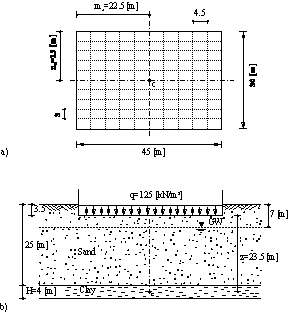Description of the problem

To verify the consolidation settlement calculated by ELPLA, the final consolidation settlement of a clay layer under a rectangular raft calculated by Graig (1978) (Example 7.2, page 186) is compared with that obtained by ELPLA.

A building is supported on a raft 45 [m] × 30 [m] is considered. The contact pressure is assumed to be uniformly distributed and equal to q = 125 [kN/m2]. The soil profile is as shown in Figure 6. The coefficient of volume change for the clay is mv = 0.35 [m/MN].

It is required to determine the final settlement under the center of the raft due to consolidation of the clay.Example 6: Consolidation settlement under a rectangular raft

Example 8: Rigid square raft on Isotropic elastic-half space mediumDescription of the problem

To verify the mathematical model of ELPLA for rigid square raft, the results of a rigid square raft obtained by other analytical solutions from Kany (1974), Fraser/ Wardle (1976), Chow (1987), Li / Dempesy (1988) and Stark (1990) (Section 5.4, page 114) are compared with those obtained by ELPLA.

The vertical displacement w [m] of a rigid square raft on Isotropic elastic-half space medium may be evaluated by ..........Example 8: Rigid square raft on Isotropic elastic-half space medium

Example 7: Consolidation settlement under a circular footing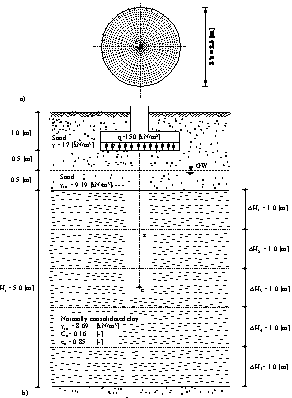Description of the problem

To verify the consolidation settlement calculated by ELPLA, the final consolidation settlement of a clay layer under a circular footing calculated by Das (1983) (Example 6.3, page 371) is compared with that obtained by ELPLA.

A circular footing 2 [m] in diameter at a depth of 1.0 [m] below the ground surface is considered as shown in Figure 7. Water table located at 1.5 [m] below the ground surface. The contact pressure under the footing is assumed to be uniformly distributed and equal to q = 150 [kN/m2]. A normally consolidated clay layer 5 [m] thick is located at a depth of 2.0 [m] below the ground surface. The soil profile is shown in the Figure. It is required to determine the final settlement under the center of the footing due to consolidation of the clay.Example 7: Consolidation settlement under a circular footing

Example 9: Rigid circular raft on Isotropic elastic-half space medium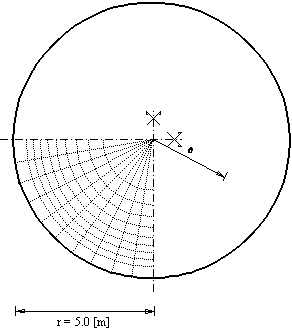Description of the problem

To verify the mathematical model of ELPLA for rigid circular raft, results of a rigid circular raft obtained by other analytical solutions from Borowicka (1939) and Stark (1990) (Section 5.2, page 106) are compared with those obtained by ELPLA.

The vertical displacement w [m] of a rigid circular raft on Isotropic elastic-half space medium may be evaluated According to Borowicka (1939).Example 9: Rigid circular raft on Isotropic elastic-half space medium

Example 10: Flexible foundation and rigid raft on layered subsoil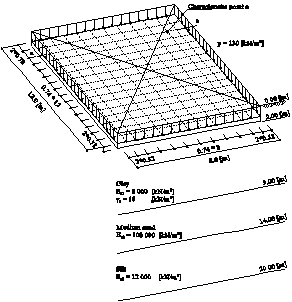Description of the problem

The definition of the characteristic point so according to Graßhoff (1955) can be used to verify the mathematical model of ELPLA for flexible foundation and rigid raft. The characteristic point of a uniformly loaded area on the surface is defined as that point of a flexible settlement so is identical with the rigid displacement wo. For a rectangular area, the characteristic point takes the coordinates ac =0.87A and bc = 0.87B, where A and B are the area sides.

Figure 12 shows a raft of dimensions 8 [m] × 12 [m] resting on three different soil layers of thicknesses 7 [m], 5 [m] and 6 [m], respectively.Example 10: Flexible foundation and rigid raft on layered subsoil

Example 11: Ultimate bearing capacity for a footing on layered subsoil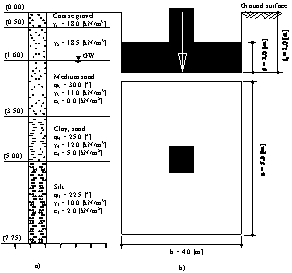Description of the problem

To verify the ultimate bearing capacity calculated by ELPLA, the results of an example in DIN 4017 (Example 2, page 9) for determining the ultimate bearing capacity of a footing on layered subsoil are compared with those obtained by ELPLA.

A rectangular footing of 4.0 [m] × 5.0 [m] on layered subsoil is considered. Footing dimensions and soil layers under the footing with soil constants are shown in Figure 14. It is required to determine the ultimate bearing capacity of the soil under the footing.Example 11: Ultimate bearing capacity for a footing on layered subsoil

Example 12: Simple assumption model for irregular raft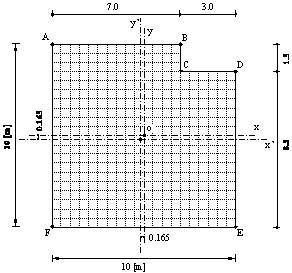Description of the problem

To verify the simple assumption model of ELPLA, the contact pressure distribution of an irregular foundation obtained by Bowles (1977) (Example 9-6, page 265) is compared with that obtained by ELPLA.

A square foundation has 10 [m] side is chosen. The foundation is subjected to a column load of 540 [kN] at the center. It is required to determine the distribution of the contact pressure when the corner is notched as shown in Figure 16.

The notch has the following properties:

Area A = 4.5 [m2]
Center of gravity from o in x-direction x! = 3.5 [m]
Center of gravity from o in y-direction y! = 4.25 [m]Example 12: Simple assumption model for irregular raft

Example 13: Main modulus of subgrade reaction ksm

Description of the problem

It is known that the modulus of subgrade reaction ks is not a soil constant but is a function of the contact pressure and settlement. It depends on foundation loads, foundation size and stratification of the subsoil. The main modulus of subgrade reaction ksm for a rectangular foundation on layered subsoil can be obtained from dividing the average contact pressure qo over the settlement so under the characteristic point on the foundation, which had been defined by Graßhoff (1955). Clearly, this procedure is valid only for rectangular foundations on a layered subsoil model. Determining the main modulus of subgrade reaction ksm for irregular foundation on an irregular subsoil model using another analysis is also possible by ELPLA.

In this example, settlement calculations at the characteristic point on the raft, using Steinbrener's formula (1934) for determining the settlement under the corner of a rectangular loaded area with the principle of superposition, are used to verify ELPLA analysis for determining the main modulus of subgrade reaction ksm. Consider the square raft in Figure 17, which has area of Af = 8×12 [m] and thickness of d = 0.6 [m].Example 13: Main modulus of subgrade reaction ksm

Example 15: Grid foundation on elastic springs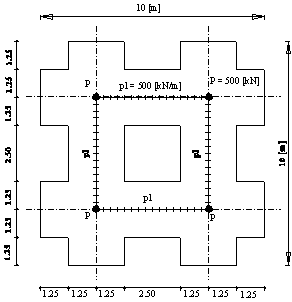Description of the problem

To verify the mathematical model of ELPLA for analyzing grid foundations, the results of grid foundation on elastic springs obtained by Szilard (1986) (Example 4.4.5, page 350) are compared with those obtained by ELPLA.

The geometry and the loads of foundation are the same as those of Szilard (1986) as shown in Figure 21. The grid has rectangular cross section of 2.5 [m] width and 0.5 [m] depth, yields Moment of Inertia I = 0.026 [m4] and Torsion modulus J = 0.091 [m4]. The parameters of grid material are Young's modulus Eb = 3×10 [kN/m] and Shear modulus Gb = 1×10 [kN/m]. Modulus of subgrade reaction of the soil is ks = 40000 [kN/m].Example 15: Grid foundation on elastic springs

Example 14: Beam foundation on elastic springs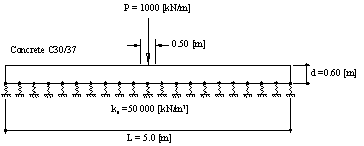Description of the problem

To verify the mathematical model of ELPLA for analyzing beam foundations, the results of beam foundation on elastic springs obtained by Rombach (2000) (Section 2.4.2, page 34) are compared with those obtained by ELPLA.

The geometry and the load of the foundation are the same as those of Rombach (2000) as shown in Figure 19. A strip foundation of thickness d = 0.60 [m] and length L = 5.0 [m] is considered.

The analysis is carried out for 1.0 [m] width stripe. The beam cross section yields Moment of Inertia I = 0.018 [m4] and Torsion modulus J = 0.045077 [m4]. The beam is subjected to a wall load of P = 1000 [kN/m] at the center.

The parameters of beam material (Concrete C30/70) are Young's modulus Eb = 3.2×107[kN/m2] and Shear modulus G = 1.3×107 [kN/m2]. Modulus of subgrade reaction of the soil is ks = 50000 [kN/m].Example 14: Beam foundation on elastic springs

Example 16: Elastic square raft on Isotropic elastic half-space soil medium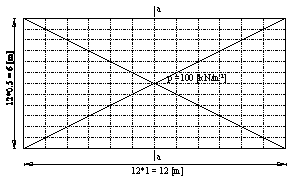Description of the problem

To verify the mathematical model of ELPLA for elastic raft, the results of an elastic raft at different relative stiffness obtained by other analytical solutions from Stark/ Majer (1988) and Borowicka (1939) are compared with those obtained by ELPLA.

A rectangular raft with sides 12 [m] and 6 [m], rests on an isotropic elastic half-space soil medium is chosen and subdivided into 12×12 elements as shown in Figure 23. The elastic properties of the raft and the soil are Es = 10000 [kN/m], Eb = 2.6×10 [kN/m], νs = 0 [-], νb = 0.15 [-]. The raft carries a uniform load of 100 [kN/m2].Example 16: Elastic square raft on Isotropic elastic half-space soil medium

Example 19: Iteration methods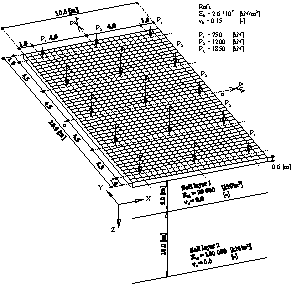Description of the problem

One of the difficulties to apply the Continuum model to practical problems is that the long computation time. Therefore, a comparison for time and accuracy required for analysis the raft by the Continuum model is carried out by the following calculation methods:

 - Modification of modulus of subgrade reaction by                iteration after Ahrens/ Winselmann (1984) (Winkle's model/ Continuum model) - Modulus of compressibility method for elastic raft on          layered soil medium after El Gendy (1998) (Solving system of linear equations by iteration) (Layered soil medium - Continuum model) - Modulus of compressibility method for elastic raft on          layered soil medium (Solving system of linear equations by elimination) (Layered soil medium - Continuum model)

To evaluate the iterative procedures used in ELPLA, consider the raft shown in Figure 30. The raft has a dimension of 10 [m] × 20 [m] and 0.6 [m] thickness.Example 19: Iteration methods

Example 20: Influence of overburden pressure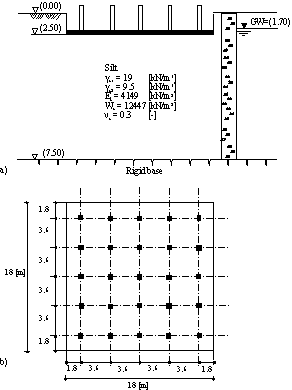Description of the problem

One of the advantages of ELPLA is that the bilinear relation of deformation for the modulus of compressibility can be taken into consideration. Therefore, an example was carried out by the Modulus of compressibility method (7) to show the influence of overburden pressure on the settlements, contact pressures and moments.

A square raft has the dimension of 18×18 [m2] under an elevated water tank was chosen as shown in the Figure.

For comparison, ELPLA was used to study the influence of overburden pressure (qv, Wv) on the values of settlements, contact pressures and moments for the following three different assumptions:

a) Without taken into consideration the influence of overburden pressure, where the modulus of compressibility for reloading Ws of the soil is taken to be equal to that of loading Es.

b) The modulus of compressibility for reloading Ws of the soil is very great (Ws = 9×108 [kN/m2]), where the settlement due to the reloading of the soil is nearly zero.

c) The modulus of compressibility for reloading Ws = 12447 [kN/m] is three times as the modulus of compressibility for loading Es = 4149 [kN/m], where the bilinear relation of deformation for the modulus of compressibility is taken into consideration.Example 20: Influence of overburden pressure

Example 17: Winkler's model and Isotropic elastic half-space soil medium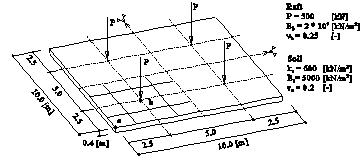Description of the problem

A simple example was carried out to verify Winkler's model and Isotropic elastic half space soil medium, by comparing ELPLA results with those of Mikhaiel (1978) (Example 34, page 189) and Henedy (1987) ( Section 3.6, page 66) (or Bazaraa (1997)).

A square raft of 0.4 [m] thickness and 10 [m] side was chosen and subdivided into 64 square elements, each has dimensions of 1.25 [m] × 1.25 [m]. The raft carries four column loads, each 500 [kN] as shown in Figure 27.Example 17: Winkler's model and Isotropic elastic half-space soil medium

Example 18: Simply supported slab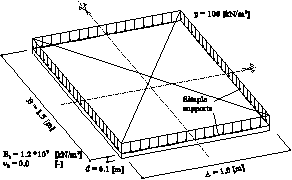Description of the problem

To examine the accuracy of the calculation of Finite elements-method and the convergence characteristics of the stiffness matrix, the maximum values of deflection w, moments mx, my and mxy of a simply supported rectangular slab are obtained at different nets of finite elements. The slab carries a uniform distributed load of p = 100 [kN/m2] as shown in Figure 28. The Young's modulus of the slab material is Eb = 1.2×10 [kN/m] and Poisson's ratio is νb = 0 [-]. The slab thickness is d = 0.1 [m].Example 18: Simply supported slab

Example 21: Influence of load geometry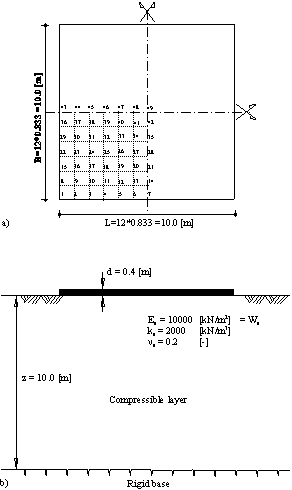Description of the problem

A simple example was carried out to show the influence of load geometry on the values of settlements and internal forces for the different subsoil models. To carry out the comparison between the different soil models, three different soil models are used to analyze the raft. The three mathematical models Simple assumption, Winkler’s and Continuum models are represented by five calculation methods.Example 21: Influence of load geometry